# “It’s turtles all the way down.” – A guide to the Basics of Data Structures

Prerequisites: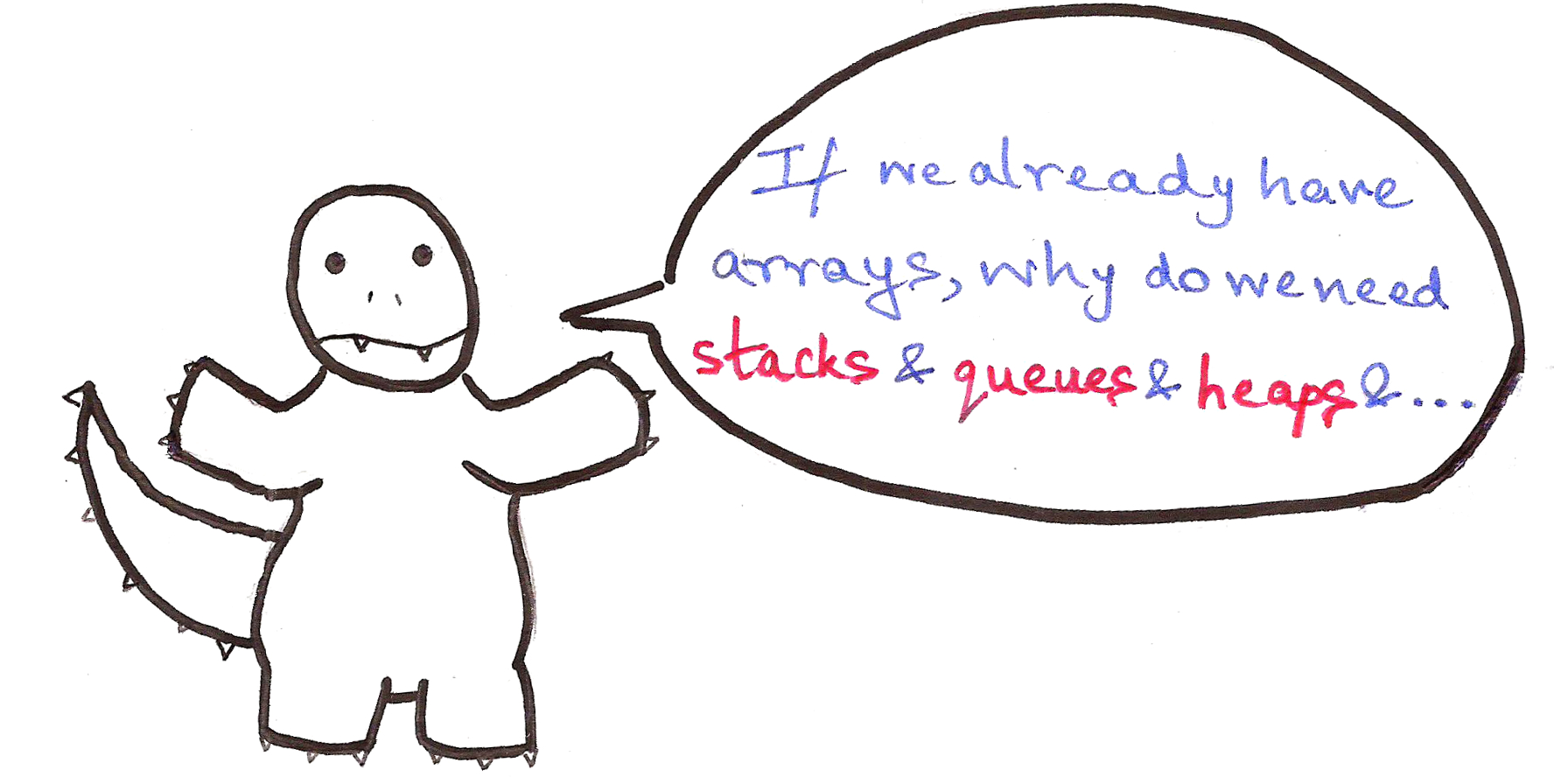Patience Algosaurus.

Data structures by themselves aren’t all that useful, but they’re indispensable when used in specific applications, like finding the shortest path between points in a map, or finding a name in a phone book with say, a billion elements (no, binary search just doesn’t cut it sometimes!).

Oh, and did I mention that they’re used just about everywhere in software systems and competitive programming?

This time, we only have two levels and a bonus, since this is an article on just the basics of data structures. Having a Mastery level just doesn’t make sense when there’s a ridiculous number of complicated data structures.

Say hello to Loopie.Loopie enjoys playing Hockey with her family. By playing, I mean…When the turtles are shucked into the goal, they are deposited back on top of the pile.

Evidently, Loopie’s family likes sliding on ice.

Notice how the first turtle added on the pile, is the first turtle to be ejected.

This is called a queue.

Similar to a real queue, the first element which was added to the list, will be the first element out. This is called a FIFO (First In First Out) structure.

Insertion and deletion operations?

Code:

```q = []

def insert(elem):
q.append(elem) #inserts elem into the end of the queue
print q

def delete():
q.pop(0) #removes 0th element of the queue
print q
```

After a fun-filled afternoon playing Hockey, Loopie is making pancakes for everyone. She places all the pancakes in a similar pile.Then serves them to the family one by one.Notice how the first pancake she made, is the last one she serves.

This is called a stack.

The first element which was added to the list, will be the last one out. This is called a LIFO (Last In First Out) structure.

Insertion and deletion operations?

Code:

```s = []

def push(elem): #insertion in a stack is called 'pushing' into a stack
s.append(elem)
print s

#deletion from a stack is called 'popping' from a stack
#pop is already a predefined function in Python for all arrays, but we'll still define it here for learning purposes as customPop()

def customPop():
s.pop(len(s)-1)
print s
```

Ever seen a density column?All the items from top to bottom, are in ascending order of their densities. What happens when you drop an object of arbitrary density into the column?￼It settles to the correct position on its own, due to difference in densities in the layers above and below it.

Heaps are something of that ilk.

A heap is a complete binary tree, meaning every parent has two children. Even though we visualize it as a heap, it is implemented through a regular array.

Also, a binary tree is always of height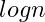, whereis the number of elements. By the way,in Computer Science is always considered to be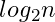by default, because we really like binary stuff.

This is a max-heap, where the fundamental heap property is that the children of any parent node, will be smaller than the parent node itself. In min-heaps, the children are always larger than the parent node.

A few basic function definitions:

```global heap
global currSize

def parent(i): #returns parent index of ith index
return i/2

def left(i): #returns left child of ith index
return 2*i

def right(i): #returns right child of ith index
return (2*i + 1)
```

Let’s tackle this part-by-part.

1) Inserting an element into a pre-existing heap

We first insert the element into the bottom of the heap, ie. last index in the array. Then we repeated apply the heap property on the index of the element till it reaches the appropriate position.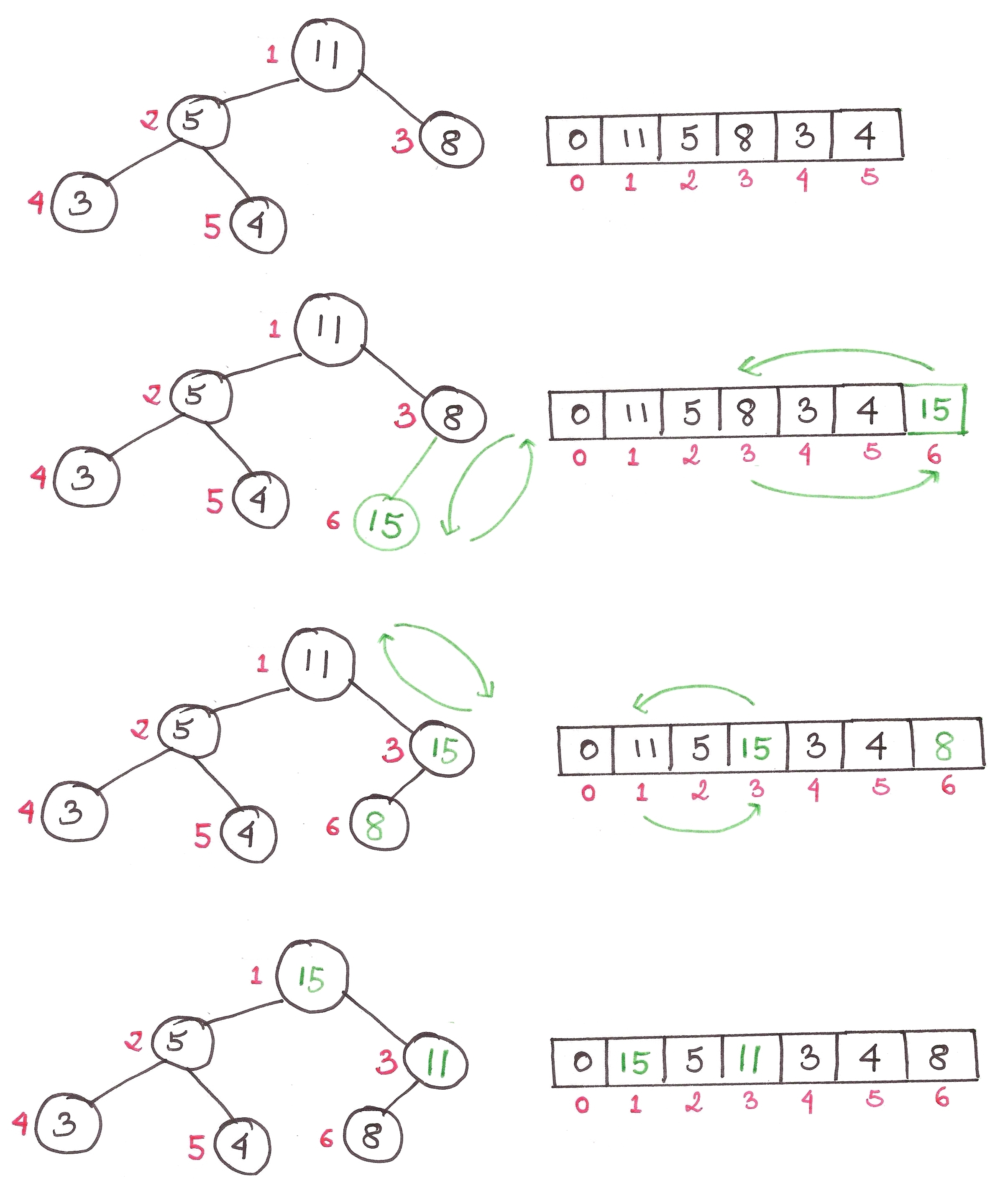The algorithm is as follows:

1. Add the element to the bottom level of the heap.
2. Compare the added element with its parent; if they are in the correct order, stop.
3. If not, swap the element with its parent and return to the previous step.

Code:

```def swap(a, b): #to swap a-th and b-th elements in heap
temp = heap[a]
heap[a] = heap[b]
heap[b] = temp

def insert(elem):
global currSize

index = len(heap)
heap.append(elem)
currSize += 1
par = parent(index)
flag = 0
while flag != 1:
if index == 1: #we have reached the root of the heap
flag = 1
elif heap[par] > elem: #if parent index is larger than index of elem, then elem has now been inserted into the right place
flag = 1
else: #swaps the parent and the index itself
swap(par, index)
index = par
par = parent(index)

print heap
```

The maximum number of times this while loop can run, is the height of the tree itself, or. Hence the time complexity is.

2) Extracting the largest element from the heap

The first element of the heap is always the largest, so we just remove that and replace the top element with the bottom one. Then we restore the heap property back to the heap, through a function called maxHeapify().1. Replace the root of the heap with the last element on the last level.
2. Compare the new root with its children; if they are in the correct order, stop.
3. If not, swap the element with one of its children and return to Step 2. (Swap with its smaller child in a min-heap and its larger child in a max-heap.)

Code:

```def extractMax():
global currSize
if currSize != 0:
maxElem = heap
heap = heap[currSize] #replaces root element with the last element
heap.pop(currSize) #deletes last element present in heap
currSize -= 1 #reduces size of heap
maxHeapify(1)
return maxElem

def maxHeapify(index):
global currSize

lar = index
l = left(index)
r = right(index)

#print heap

#finds the larger child of the index; if larger child exists, swaps it with the index
if l <= currSize and heap[l] > heap[lar]:
lar = l
if r <= currSize and heap[r] > heap[lar]:
lar = r
if lar != index:
swap(index, lar)
maxHeapify(lar)
```

Again, the maximum number of times maxHeapify() can be executed, is the height of the tree itself, or. Hence the time complexity is.

3) How to make a heap out of any random array

Okay, so there’s two ways to go about it. The first way is to just repeatedly insert every element into the previously empty heap.

This is easy, but relatively inefficient. The time complexity of this comes out to be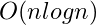because of anfunction being runtimes.

There’s a better, more efficient way of doing this, where we simply maxHeapify() every ‘sub-heap’ from theelement back to the 1st one.

This runs at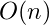complexity and it’s beyond the scope of this article to prove why. Just understand that any element past the halfway point in a binary tree, will have no children and that most of the ‘sub-heaps’ formed will be of height less than.

Code:

```def buildHeap():
global currSize
for i in range(currSize/2, 0, -1): #third argument in range() shows increment factor, here -1
print heap
maxHeapify(i)
currSize = len(heap)-1
```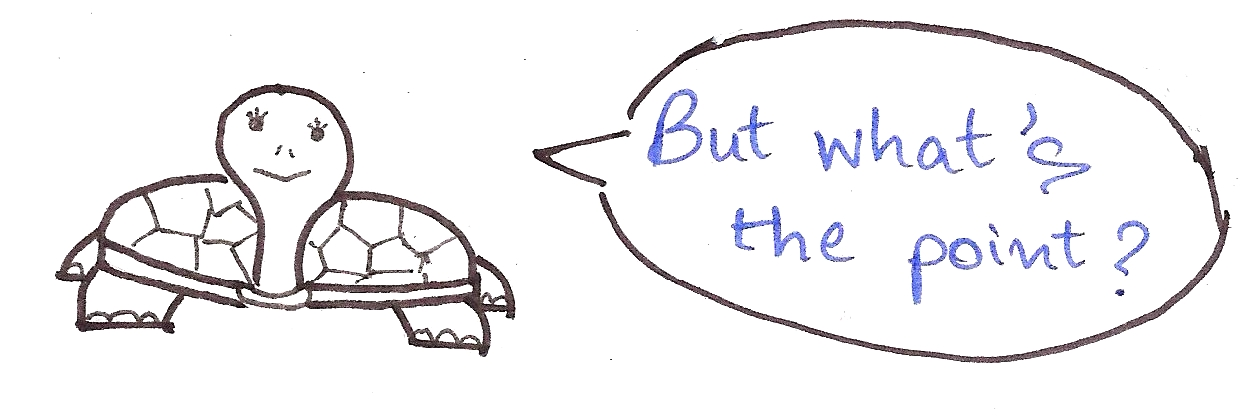Ah, we’ve been leading up to this question this entire time.

Heaps are used to implement an efficient sort of sort, unsurprisingly called, the Heapsort.

Unlike the sorely inefficient Insertion Sort and Bubble Sort with their measly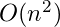complexities, Heapsort runs intime, beating them to the dust.

It’s not even complicated, just keep extracting the largest element from the heap till the heap is empty, placing them sequentially at back of the array where the heap is stored.

Code:

```def heapSort():
for i in range(1, len(heap)):
print heap
heap.insert(len(heap)-i, extractMax()) #inserting the greatest element at the back of the array
currSize = len(heap)-1
```

To tie it all together, I’ve written a few lines of helper code to input elements into the heap and try out all the functions. Check it out right here. Oh, and for all the people who are acquainted with classes in Python, I’ve also written a Heap class here.

Voila! Wasn’t that easy? Here’s a partying Loopie just for coming this far.We also use heaps in the form of priority queues to find the shortest path between points in a graph using Dijkstra’s Algorithm, but that’s a post for another day.

Loopie wants to teach her baby turtles how to identify shapes and colours, so she brings home a large number of pieces of different colours.This took them a lot of time, as well as confusion.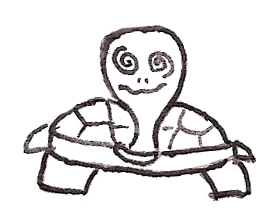So she gets another toy to make the process easier.This was easier because the babies already knew that the pieces were categorized according to shape. What if we labeled each of the poles as follows?The babies would just have to check for the pole number, then look through a far smaller number of pieces on the pole.

Now imagine one pole for every combination of colour and shape possible.

Let the pole number be calculated as follows:

purple triangle:
p+u+r+t+r+i = 16+21+18+20+18+9 = Pole #102

red rectangle:
r+e+d+r+e+c = 18+5+4+18+5+3 = Pole #53

etc.

We know that 6*26 = 156 combinations are possible (why?), so we’ll have 156 poles in total.

Let’s call this formula to calculate pole numbers, the hash function.

In code:

```def hashFunc(piece):
words = piece.split(" ") #splitting string into words
colour = words
shape = words
poleNum = 0
for i in range(0, 3):
poleNum += ord(colour[i]) - 96
poleNum += ord(shape[i]) - 96
return poleNum
```

If we ever need to finish where ‘pink square’ is kept, we just use

`hashFunc('pink square')`

and check the pole number, which happens to be pole #96.This is an example of a hash table, where the location of an element is stored in terms of a hash function. The poles here are analogous to buckets in proper terminology.

This makes time taken to search for a particular element independent of the total number of elements, ie.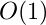time.

Let this sink in. Searching in a hash table can be done in constant time.

Wow.

What if we’re searching for a ‘dreary-blue rectangle’, assuming a colour called ‘dreary-blue’ exists?

`hashFunc('dreary-blue rectangle')`

returns pole #53, which clashes with the pole number for ‘red rectangle’. This is called a collision.

How do we resolve it?

We use a method called separate chaining, which is a fancy way of saying every bucket consists of a list, and we simply search through the list if we ever find multiple entries.

Here, we’ll just put the dreary-blue rectangle on top of the red rectangle, and just pick either one whenever we need to.

The key in any good hash table, is to choose the appropriate hash function for the data. This is unarguably the most important thing in making a hash table, so people spend a lot of time on designing a good hash function for their purpose.

In a good hash table, no bucket should have more than 2-3 entries. If there are more, then the hashing isn’t working well, and we need to change the hash function.Searching is independent of the number of elements for god’s sake. We can use hash tables for just about anything involving a gigantic number of elements, like database entries or phone books.

We also use hash functions in searching for strings or sub-strings in large collections of texts using the Rabin-Karp algorithm. This is useful for detecting plagiarism in academic papers by comparing them against source material. Again, a post for another day!I plan on writing more on advanced data structures like Fibonacci Heaps and Segment Trees and their uses, so subscribe to Algosaurus for updates!

I hope you found this informal guide to the basics of data structures useful. If you liked it, want to insult me, or if you want to talk about dinosaurs or whatever, shoot me a mail at rawrr@algosaur.us

Acknowledgements:
Introduction to Algorithms – Cormen, Leiserson, Rivest, and Stein (pages 151 – 161)

# “To iterate is human, to recurse, divine.” – A guide to RecursionPrerequisites:

• Logical progression of thought
• Some mathematical aptitude
• Basic knowledge of functions

Recursion is possibly one of the most challenging concepts in Computer Science to get the hang of, and something which has fascinated me for a very long time. Sure, we all know the textbook definition:

“Recursion is a method where the solution to a problem depends on the solutions to smaller instances of the same problem.”

But it still takes an “Aha! Moment” to get an intuitive grasp on it, which is why I’ll finally take a stab at it.

As usual, we have three levels, so switch to them as you see fit:

Algosaurus coincidentally happens to be part of an obscure death metal band you’ve never heard of. He goes to a concert venue with a poorly-designed audio system and starts off with his smash-hit song “Rock Tore My World Apart”. He growls into the mic as he descends into the chorus and…

…is rudely interrupted by a loud screeching sound from the amplifier.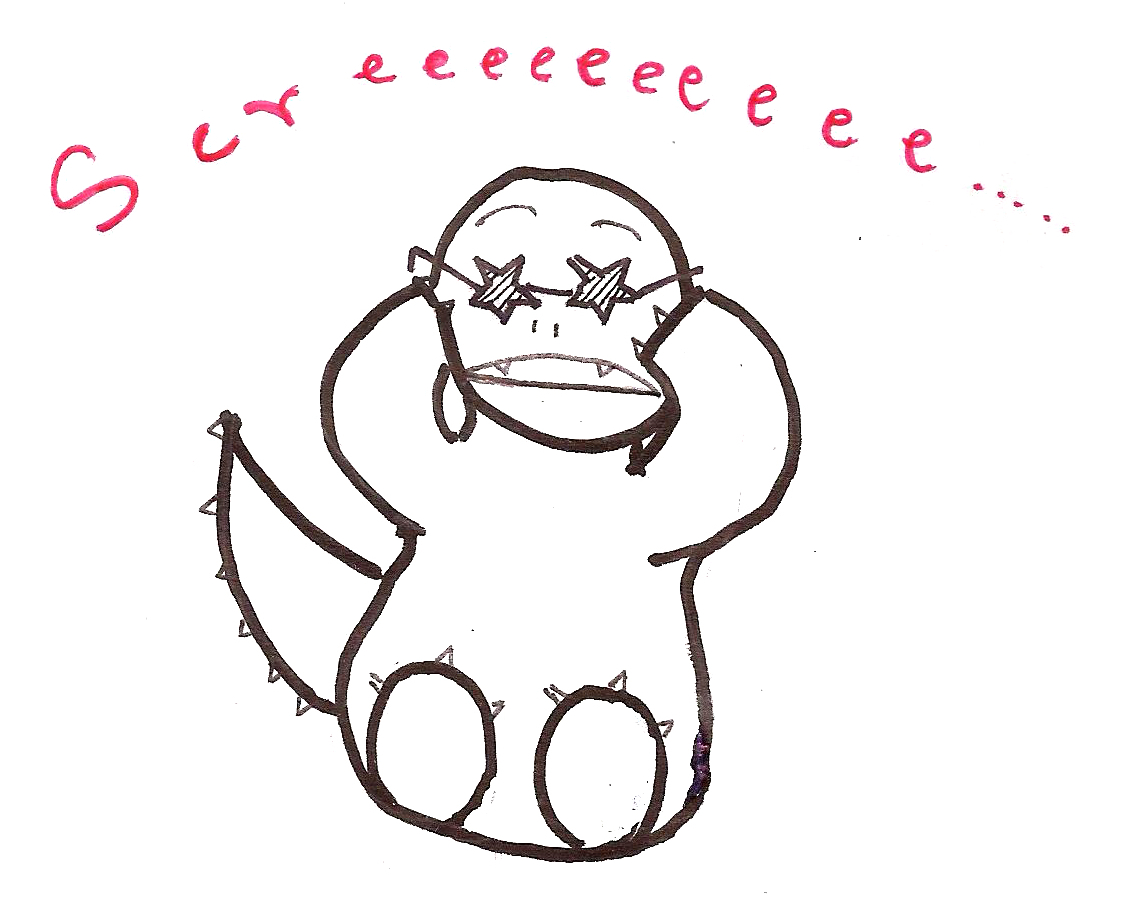What just happened was a feedback loop. While Algosaurus was singing (growling?) his heart out, his mic picked up the output of the surrounding amplifiers, and fed it right back into the input.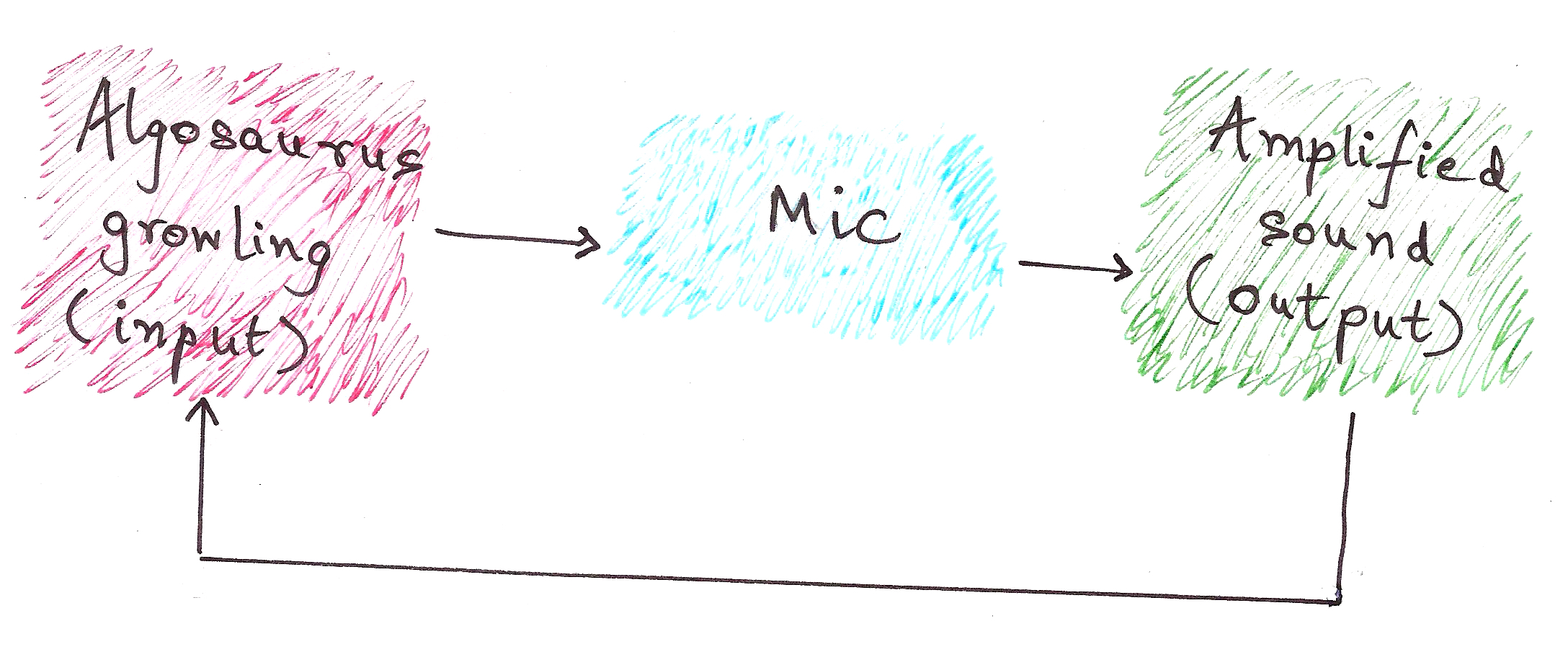Although the above example doesn’t reduce to a meaningful output, rather it results in a stack overflow (the screeching sound), this is the essential idea of recursion.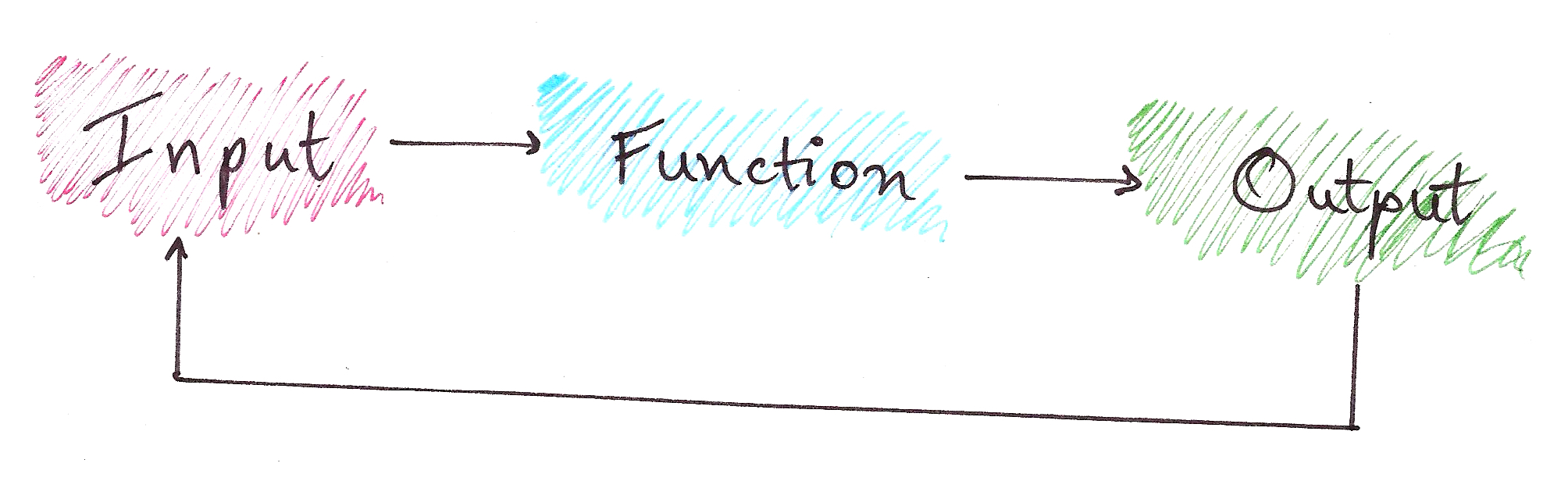In recursion, we have:

Problem
|
Sub-problem (which is Problem with a smaller input)
|
Base Case (a Problem with a constant output)

Confused by the jargon?So was I.

No article on recursion is complete without talking about Fibonacci Numbers.

Fibonacci series: 0, 1, 1, 2, 3, 5, 8, 13, 21…

You’ll notice that the nth Fibonacci number, after n = 0 and n = 1, is the sum of the previous two Fibonacci numbers.

Namely,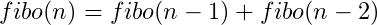Notice how this is a recursive definition? Let’s break it down into a recursion tree.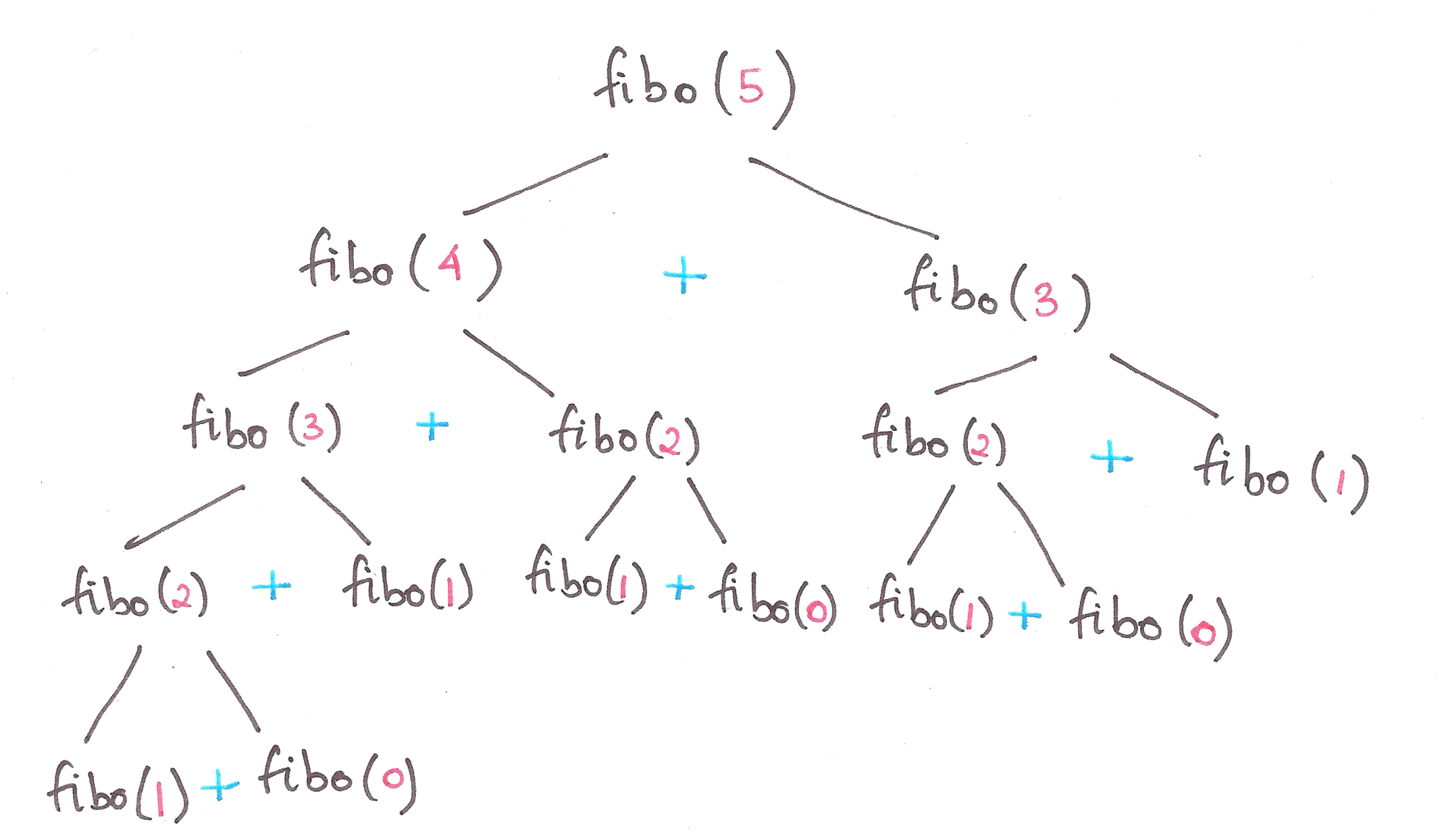So, let’s define Fibonacci numbers in terms of Problems and Base Cases.

Problem:|
Sub-problem(s):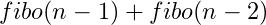|
Constant Base Case: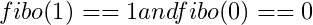This is also known as the divide-and-conquer paradigm of algorithms, where we segregate a problem into smaller sub-problems, and finally into a simple base case.

Final code for Fibonacci numbers?

```def fibo(n):
if n == 0:
result = 0 #Base case
elif n == 1:
result = 1 #Base case
else:
result = fibo(n-1) + fibo(n-2) #Feeding the 'output' right back into the input function
return result
```Lo and behold, you just learnt recursion.

Let’s take another classic example to hit the point home.

Once upon a time, in the Cretaceous Era, there lived a family of Algosauri in a land far, far away. Baby Algosaurus had a toy with 3 rods and 7 discs.His Dad challenged him to a game, where the objective was to transfer all the discs to another rod, obeying the following rules:

1. Only one disk may be moved at a time.
2. Only the uppermost disk from one rod can be moved at once.
3. It can be put on another rod, only if that rod is empty or if the uppermost disk of that rod is larger than the disc being moved.

How did Baby Algosaurus do it?

Let’s assume there to be n number of discs, and we’ll be moving the tower from source to target using the helper rod:

For n = 1: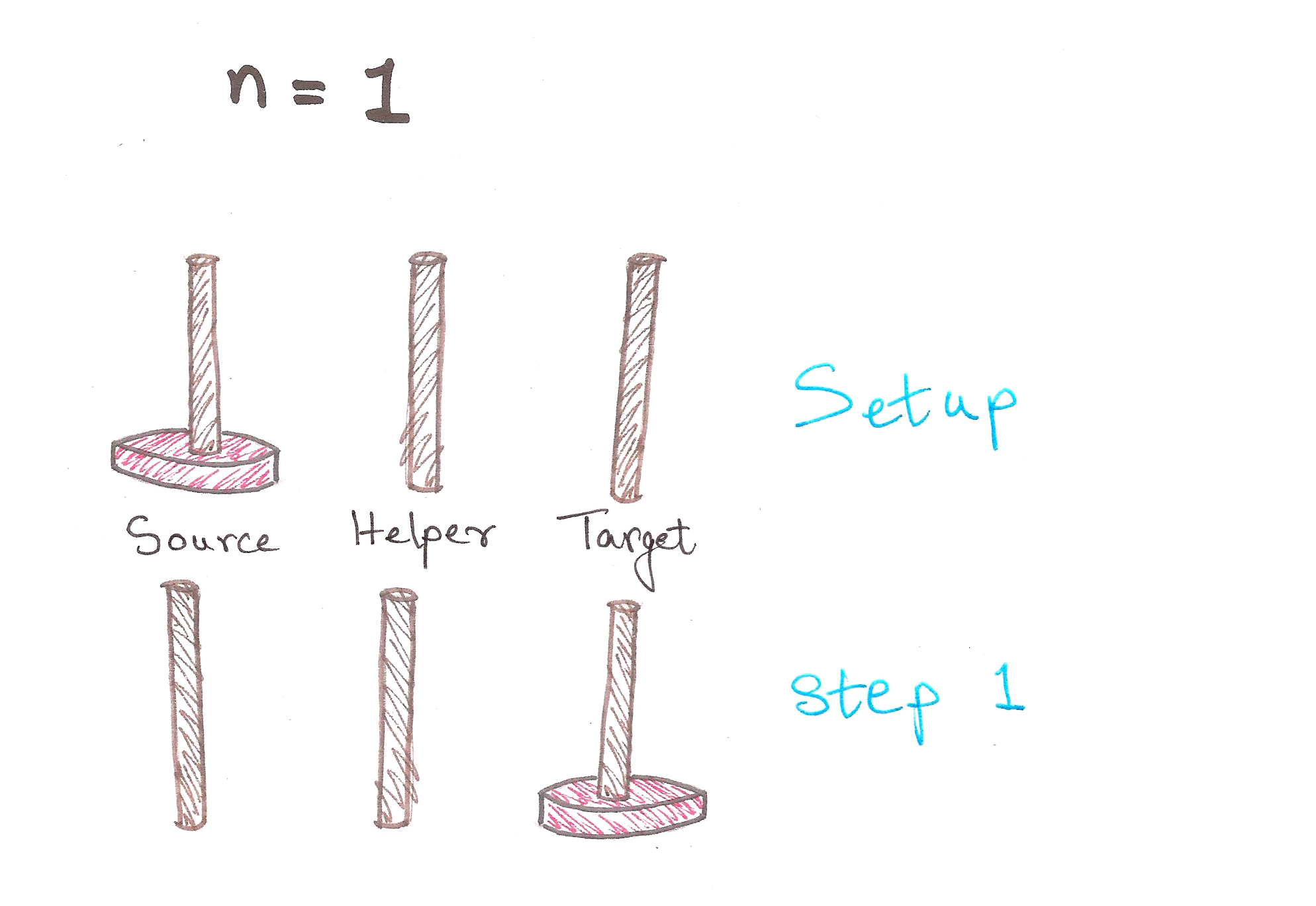1 step. Easy enough.

For n = 2: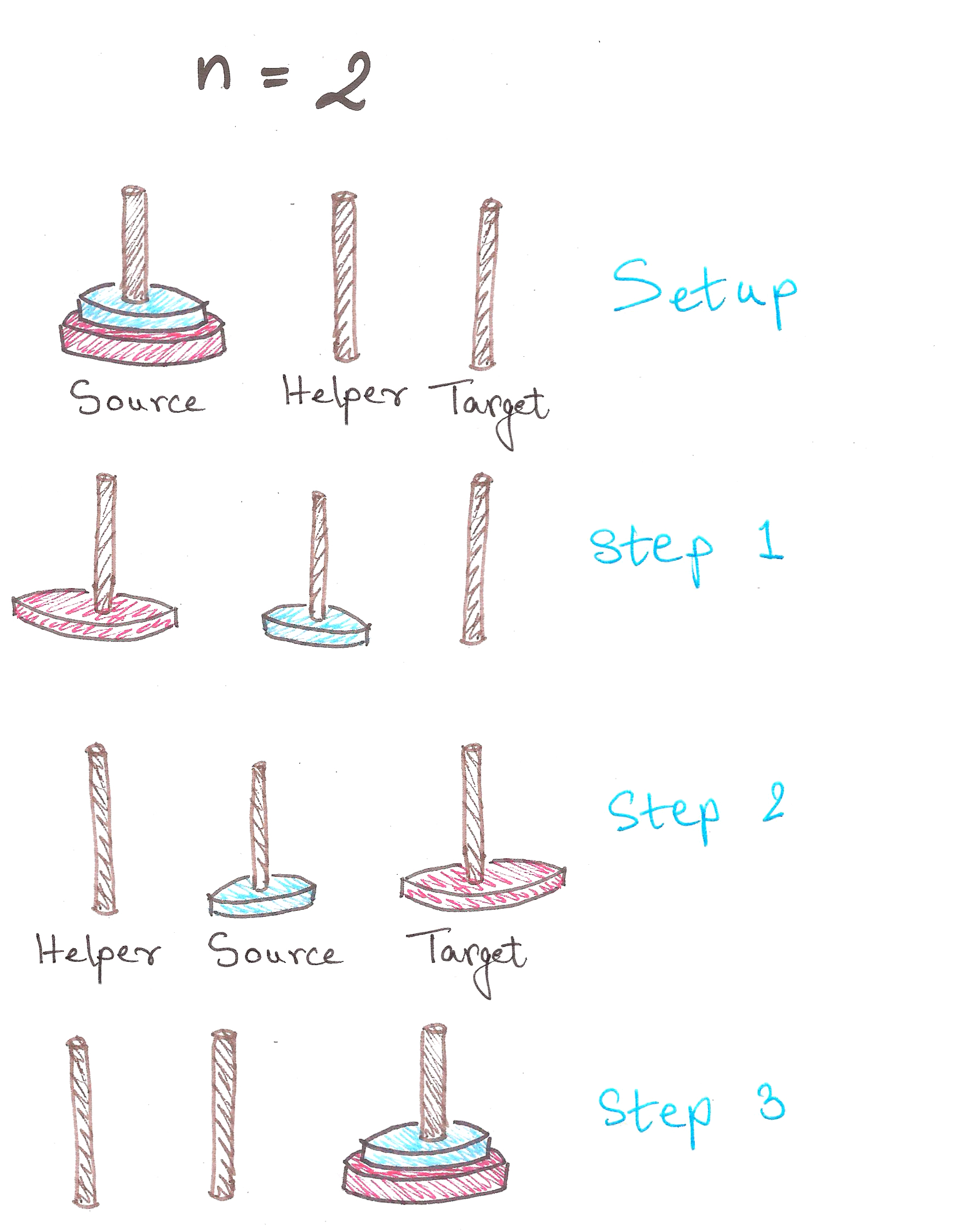3 steps. We’re moving along now.
In Step 1, notice how after we shift the 1st disc to Helper, we have a tower of n – 1 = 1 disc, on Helper.

For n = 3:And we’re done in 7 steps!
Observe that in Step 3, we have a tower of n – 1 = 2 discs, on Helper now. Notice anything similar to the previous case?

In case you haven’t noticed…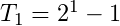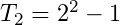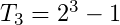In fact, we can prove by mathematical induction, that the least number of moves required to solve a tower of n discs is:Back? Good.

We now need to solve the problem for n = 7. We can of course, figure it out by hand as above, but what’re we learning recursion for then?

A quick recap of the divide-and-conquer method:

Problem
|
Sub-problem (which is a Problem with a smaller input)
|
Base Case (a Problem with a constant output)

So here, in the Tower of Hanoi problem (yeah, that’s the official name), we have:

The general rule for moving towers:Problem: Moving tower of n discs from Source to Target
|
Sub-Problem: Moving tower of n – 1 discs from Source to Helper, then moving last disc to Target
|
Base Case: Moving tower of n = 1

Great. Let’s finally translate this into code.

```def hanoi(n, source, helper, target):
if n > 0:
hanoi(n - 1, source, target, helper)    #moving tower of size n - 1 to helper:
#moving disk from source peg to target peg
if source:    #checks whether source is empty or not
target.append(source.pop())    #appends the last disc from source, to target
hanoi(n - 1, helper, source, target)    #moving tower of size n-1 from helper to target

source = [4,3,2,1]
target = []
helper = []
hanoi(len(source),source,helper,target)

print source, helper, target
```Feel free to go through this entire section once more to digest it, because it’s pretty mentally intensive if you aren’t yet used to thinking this way.

Proof by induction:

The base cases for this problem are pretty simple…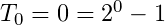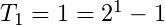Let’s assume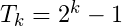to be true for now.

To prove: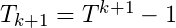is true.

So we have,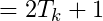Self-similarity is symmetry across scale. It implies recursion, pattern inside of pattern. Monstrous shapes like the Koch Curve display self-similarity because they look exactly the same, even under high magnification. Self-similarity is an easily recognizable quality. It’s images are everywhere in the culture: in the infinitely deep reflection of a person standing between two mirrors, or in the cartoon notion of a fish eating a smaller fish eating a smaller fish eating a smaller fish.

– Chaos – The Amazing Science of the Unpredictable, James Gleick

In 1976, a biologist named Robert May published a seminal paper on the population of animals. This paper would soon create a storm, and not just in biology.

Let’s take a look at what it was all about.

May proposed that variation in population of animals could be modeled by a simple equation, formally called the logistic equation: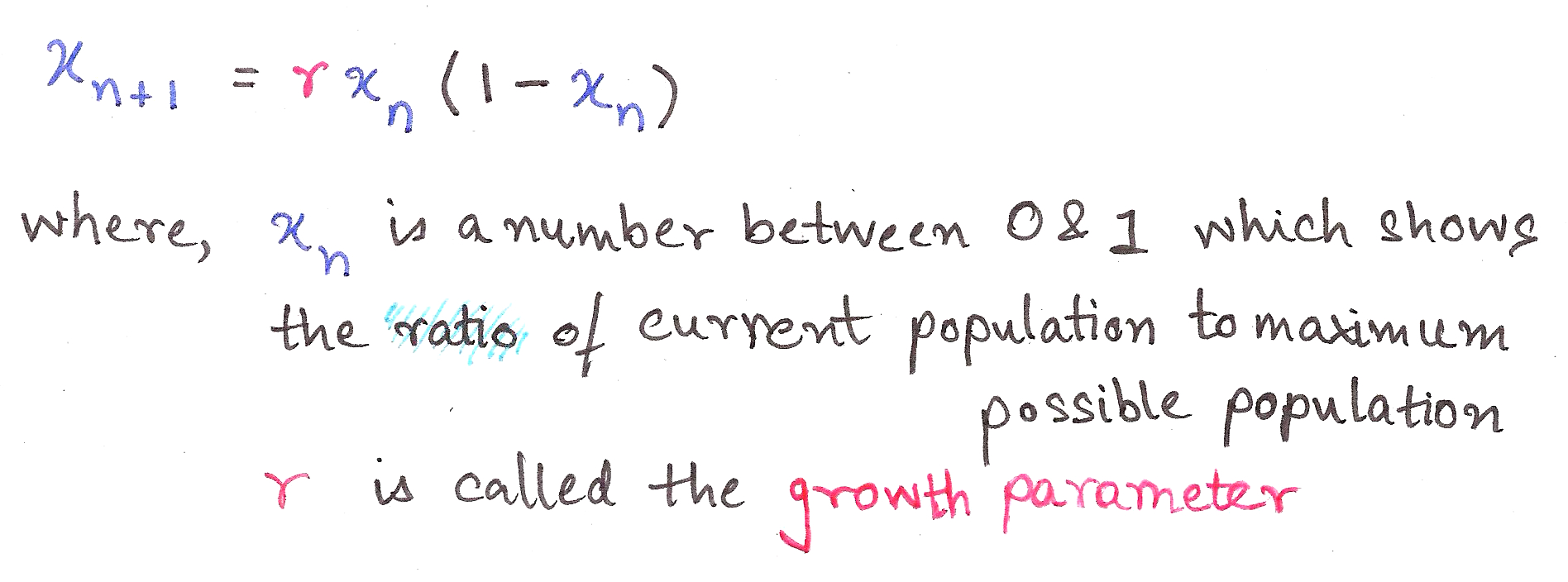This is a recurrence relation, an equation which recursively spits out values as a function of its previous values, ie. takes the output and shoves it back into the input.

It’s such a cute equation, isn’t it? Who’d guess that its interpretation could transform into *this*…This is called a bifurcation diagram, and is also a kind of fractal. It shows the values the population of the species evaluates to finally (as time tends to infinity), depending on the value of r, the growth parameter.

Let’s dissect this.

r between 0 and 1 – Population dies out
r between 1 and 3 – Population converges to a single value
r between 3 and 3.44 – Population oscillates between two values
r between 3.44 and 3.54 – Oscillates between four values
r between 3.54 and 3.56 – Oscillates between 8 values

All of the above are pretty much independent of initial conditions, ie. the initial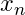we take.

Chaos.

Slight differences in initial conditions result in dramatic changes in population, with no particular order. (In science-y language, ‘Extreme sensitivity to initial conditions’)

This particular paper was path-breaking because it showed how Chaos Theory and fractals aren’t obscure theoretical constructs; they’re things which explain real-life phenomena and its glorious imperfections.

This is a bit beyond the scope of this article, but it goes to show how wide-ranging a role does Recursion play, not just in computer science, but in nature itself.I hope you now have enough a grasp on thinking recursively to understand algorithms like Quick Sort and Merge Sort, and attempt a few basic ad-hoc problems from Codechef et al.

If you are just as fascinated as I am about recursion, then you might enjoy reading about the following:
Fractals
Chaos Theory
Infinite Series and Loops
Feedback loops in Op-amps (they’re an essential part of electronics)

I plan on writing more about the above topics, so do subscribe to Algosaurus for updates.

If you liked this guide, want to insult me, or if you want to talk about dinosaurs or whatever, shoot me a mail at rawrr@algosaur.us

Acknowledgements:
http://www.python-course.eu/towers_of_hanoi.php
https://en.wikipedia.org/wiki/Logistic_map